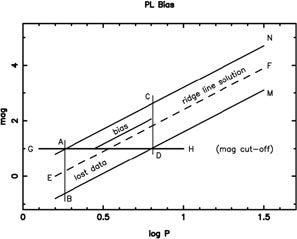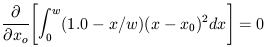### 12. APPENDIX A: MAGNITUDE-LIMITED BIAS

We present here an analytic derivation of the bias introduced into the PL fits imposed by magnitude-limited cuts on extragalactic Cepheid samples. We note that in Section 3.4, the application of short-period cuts to the observed PL relations (to compensate for this bias) resulted in slightly increased moduli. Depending on the sample size and its period distribution, these empirical corrections ranged from <1% up to 4% in distance. We now provide an analytic solution to reinforce our understanding of the degree and direction of this bias.

Consider Figure A1, which is meant to represent a uniform distribution of Cepheids defining a period-luminosity relation of finite width CD. It is clear that the dataset defined by the parallelogram ABMN will be unbiased with respect to a fit EF (dashed ridge line), where EF has the predefined slope. It is fit to the data distribution within ABMN using least squares, assuming that all of the variance is in the vertical direction, and that errors on the periods are negligible.Figure A1. Illustration of bias due to a magnitude cutoff in the Cepheid period-luminosity relation. See text for details.

Now if a magnitude limiting cut-off to the Cepheid data is imposed (by line GH), it is clear that an asymmetry in the data distribution will be introduced with a bias in the fitted zero point ensuing: the region ACD is uncompensated for by the exclusion of its complementary region ADB. Of course the full bias introduced by ACD will depend on the relative numbers of stars in this section as compared to those in the unbiased area. However, if the section ACD is uniformly populated it can be shown that a fixed-slope solution (to that portion only) fit by least squares would introduce a bias toward brighter magnitudes by an amountm = w/6, where w is the full magnitude width (measured at fixed period) of the instability strip.

The minimization of the least-squares conditioninggives xo = w / 3 such that the difference between the biased solution xo and the unbiased solution at w / 2 is xo - w / 2 = - w / 6

For the reddening-free W-PL relation, where the Key Project fitting is done, the intrinsic scatter in the relation is= 0.08 mag (Udalski et al. 1999) giving w = 4 ×~ 0.3 mag, and a predicted (maximum) bias of 0.05 mag, or less than about 3% in distance. This bias term will of course be diluted in direct proportion with the relative numbers of stars outside of the biased zone. This is in complete agreement with the quantitative corrections found in the main text, where the typical correction is +0.02 mag, with the largest correction leading to an increase of 4% in distance.